# Hexadecimal Data representation 2 Objectives Convert positive denary

• Slides: 20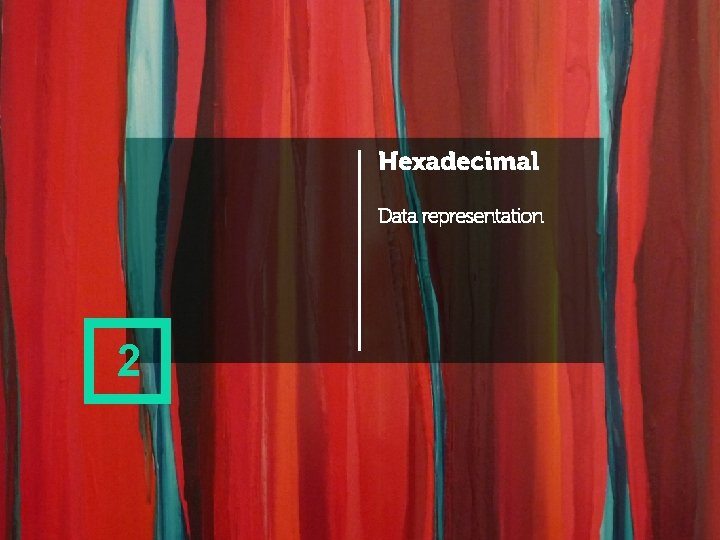Hexadecimal Data representation 2Objectives • Convert positive denary whole numbers (0 -65, 535) into 2 -digit hexadecimal numbers and vice versa • Convert between 16 -bit binary and hexadecimal equivalents of the same number • Explain the reasons for using hexadecimal numbers to represent numbers • Represent numbers stored in registers and main memory as hexadecimal • Identify current uses of hexadecimal numbers in computingHexadecimal Data representation Binary to denary conversion • Practise converting binary to denary and back again • Convert the following: 0 1 14 105 0 0 1 1 0 Which is odd and which is even? How can you tell?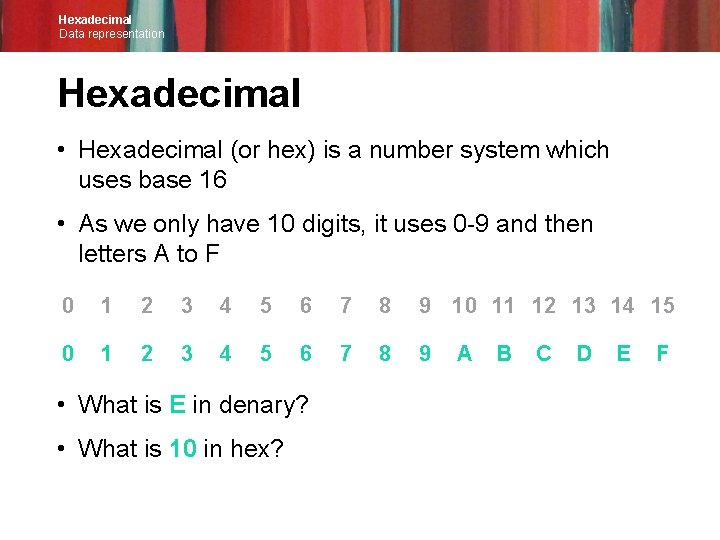Hexadecimal Data representation Hexadecimal • Hexadecimal (or hex) is a number system which uses base 16 • As we only have 10 digits, it uses 0 -9 and then letters A to F 0 1 2 3 4 5 6 7 8 9 10 11 12 13 14 15 0 1 2 3 4 5 6 7 8 9 • What is E in denary? • What is 10 in hex? A B C D E F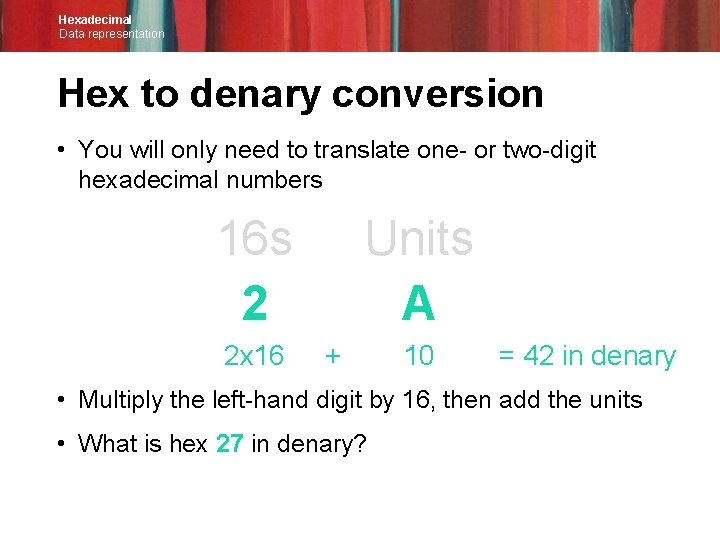Hexadecimal Data representation Hex to denary conversion • You will only need to translate one- or two-digit hexadecimal numbers 16 s 2 2 x 16 Units A + 10 = 42 in denary • Multiply the left-hand digit by 16, then add the units • What is hex 27 in denary?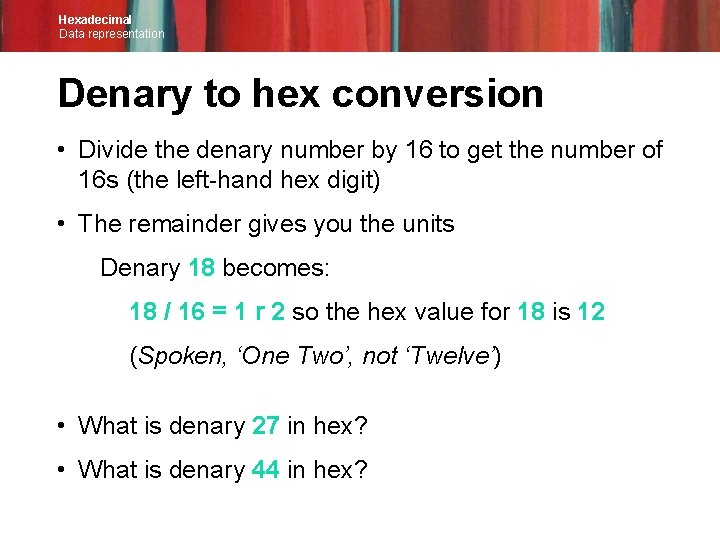Hexadecimal Data representation Denary to hex conversion • Divide the denary number by 16 to get the number of 16 s (the left-hand hex digit) • The remainder gives you the units Denary 18 becomes: 18 / 16 = 1 r 2 so the hex value for 18 is 12 (Spoken, ‘One Two’, not ‘Twelve’) • What is denary 27 in hex? • What is denary 44 in hex?Hexadecimal Data representation Worksheet 2 • Complete Task 1 Question 1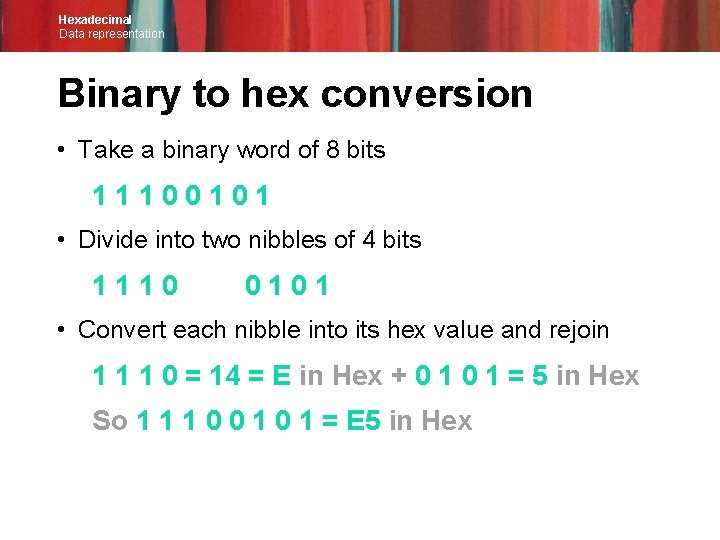Hexadecimal Data representation Binary to hex conversion • Take a binary word of 8 bits 11100101 • Divide into two nibbles of 4 bits 1110 0101 • Convert each nibble into its hex value and rejoin 1 1 1 0 = 14 = E in Hex + 0 1 = 5 in Hex So 1 1 1 0 0 1 = E 5 in HexHexadecimal Data representation Hex to binary conversion • What is 3 B in hex? Split the two hex characters 3 = 0011 in binary and B = 1011 So 3 B = 0011 1011 in binary What is hex 21 in binary? What is hex A 5 in binary?Hexadecimal Data representation Worksheet 2 • Complete Task 1 Question 2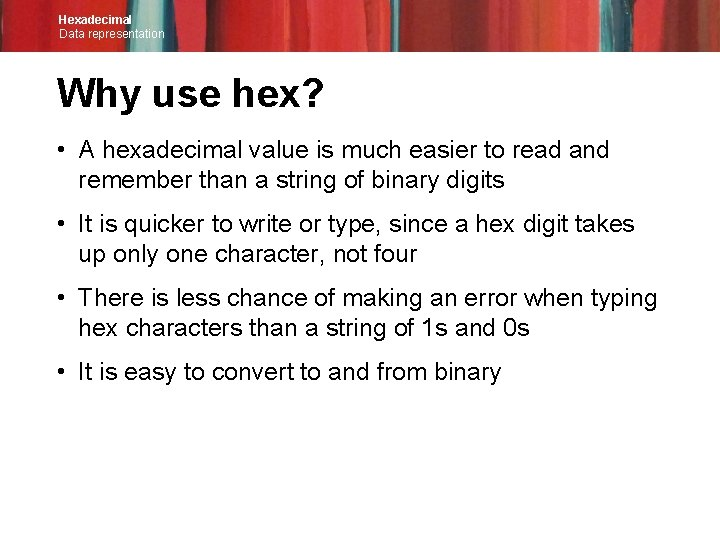Hexadecimal Data representation Why use hex? • A hexadecimal value is much easier to read and remember than a string of binary digits • It is quicker to write or type, since a hex digit takes up only one character, not four • There is less chance of making an error when typing hex characters than a string of 1 s and 0 s • It is easy to convert to and from binaryHexadecimal Data representation Using hexadecimal • One common use of hexadecimal numbers is to set colours on web pages • A colour is defined by the amount of red, green and blue it contains: e. g. = 3 D 7 EB 5 • Each of these RGB values can be in the range 0 to 255 (decimal) or 00 to FF (hexadecimal) • This gives a possible 256 x 256 colours, which is more than 16 million • How many bits are used to represent a single colour?Hexadecimal Data representation Defining colours in website design • HTML (Hyper. Text Markup Language) is used to create web pages h 1 {color: #ffa 347; }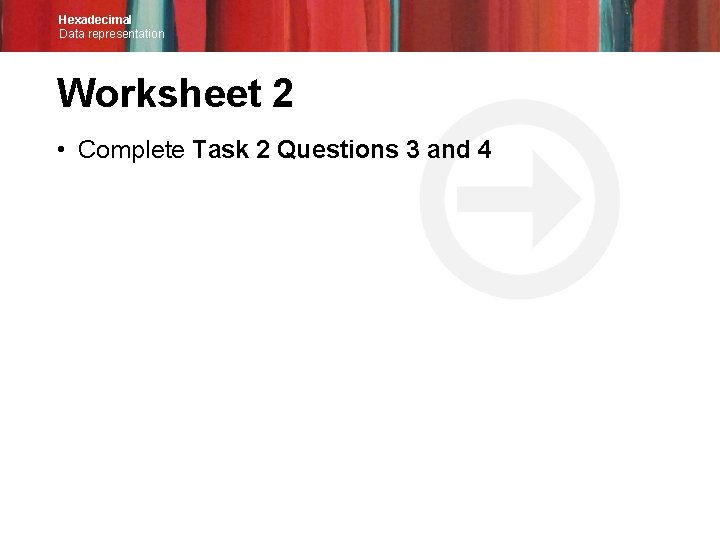Hexadecimal Data representation Worksheet 2 • Complete Task 2 Questions 3 and 4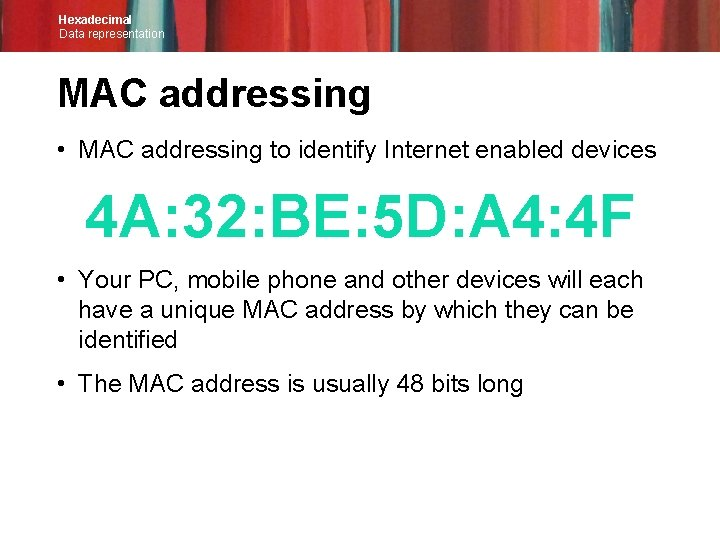Hexadecimal Data representation MAC addressing • MAC addressing to identify Internet enabled devices 4 A: 32: BE: 5 D: A 4: 4 F • Your PC, mobile phone and other devices will each have a unique MAC address by which they can be identified • The MAC address is usually 48 bits long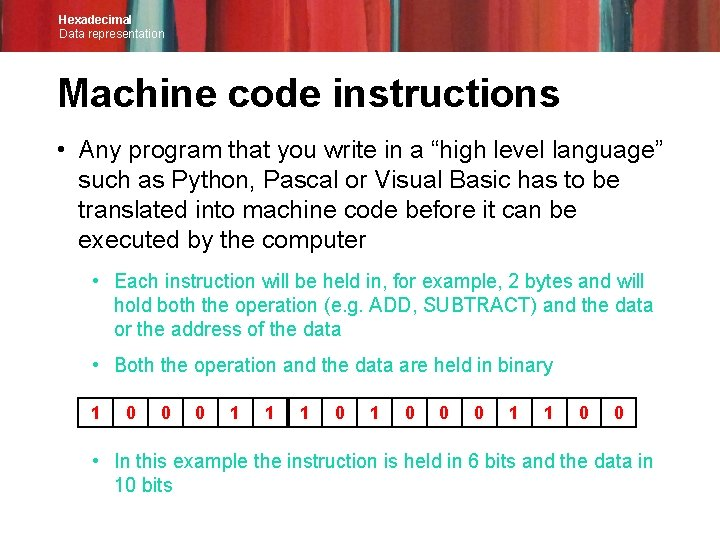Hexadecimal Data representation Machine code instructions • Any program that you write in a “high level language” such as Python, Pascal or Visual Basic has to be translated into machine code before it can be executed by the computer • Each instruction will be held in, for example, 2 bytes and will hold both the operation (e. g. ADD, SUBTRACT) and the data or the address of the data • Both the operation and the data are held in binary • 1 0 0 0 1 1 0 0 • In this example the instruction is held in 6 bits and the data in 10 bits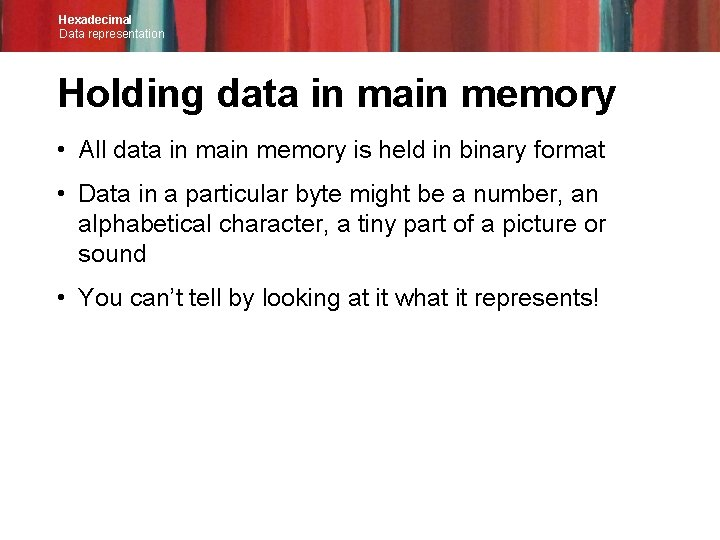Hexadecimal Data representation Holding data in main memory • All data in main memory is held in binary format • Data in a particular byte might be a number, an alphabetical character, a tiny part of a picture or sound • You can’t tell by looking at it what it represents!Hexadecimal Data representation Using hex for debugging • Programmers sometimes need to examine what is going on in a program by looking at the contents of memory • Printed out in binary, it might look something like this: 00011001 111100011001 1010 01001100 00111101 10001110 etc. • It is much easier to debug or find a particular value if it printed out in hex! 19 99 F 1 19 AA 4 C 3 D 8 E etc.Hexadecimal Data representation Worksheet 2 • Now complete Task 3, Questions 5 and 6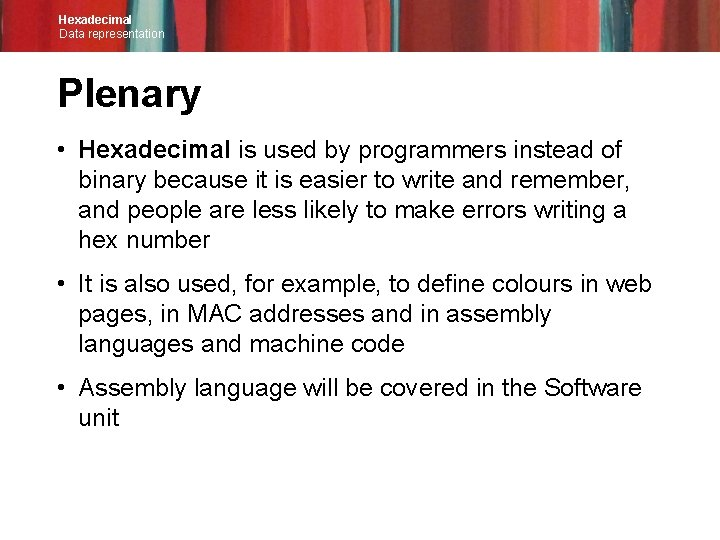Hexadecimal Data representation Plenary • Hexadecimal is used by programmers instead of binary because it is easier to write and remember, and people are less likely to make errors writing a hex number • It is also used, for example, to define colours in web pages, in MAC addresses and in assembly languages and machine code • Assembly language will be covered in the Software unit